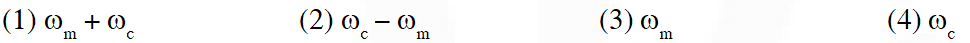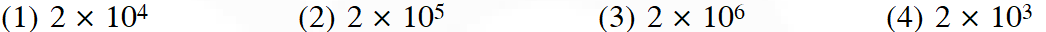Most Affordable JEE | NEET | 8,9,10 Preparation by Kota's Top IITian Doctor Faculties

# Communication System - JEE Main Previous Year Questions with SolutionsJEE Main Previous Year Question of Physics with Solutions are available here. Practicing JEE Main Previous Year Papers Questions of Physics will help all the JEE aspirants in realizing the question pattern as well as help in analyzing their weak & strong areas. Get detailed Class 11th &12th Physics Notes to prepare for Boards as well as competitive exams like IIT JEE, NEET etc. eSaral helps the students in clearing and understanding each topic in a better way. eSaral is providing complete chapter-wise notes of Class 11th and 12th both for all subjects. Besides this, eSaral also offers NCERT Solutions, Previous year questions for JEE Main and Advance, Practice questions, Test Series for JEE Main, JEE Advanced and NEET, Important questions of Physics, Chemistry, Math, and Biology and many more. Download eSaral app for free study material and video tutorials.   Simulator Previous Years AIEEE/JEE Mains Questions
Q. This questions has Statement-1 and Statement-2. Of the four choice given after the statements, choose the one that best describes the two statements. Statement-1 : Sky wave signals are used for long distance radio communication. These signals are in general, less stable than ground wave signals. Statement-2 : The state of ionosphere varies from hour to hour, day to day and season to season. (1) Statement-1 is true, Statement-2 is true, Statement-2 is the correct explanation of statement-1 (2) Statement-1 is true, Statement-2 is true, Statrment-2 is not the correct explanation of Statement-1 (3) Statement-1 is false, Statement-2 is true (4) Statement-1 is true, Statement-2 is false [AIEEE-2011]
Ans. (2)
Q. A radar has a power of 1 kW and is operating at a frequency of 10 GHz. It is located on a mountain top of height 500 m. The maximum distance up to which it can detect object located on the surface of the earth (Radius of earth $\left.=6.4 \times 10^{6} \mathrm{m}\right)$ is : (1) 40 km (2) 64 (3) 80 km (4) 16km [AIEEE-2012]
Ans. (3) $\mathrm{d}=\sqrt{2 \mathrm{Rh}}=\sqrt{2 \times 6400 \times 0.5}=80 \mathrm{km}$
Q. A diode detector is used to detect an amplitude modulated wave of 60% modulation by using a condenser of capacity 250 pico farad in parallel with a load resistance 100 kilo ohm. Find the maximum modulated frequency which could be detected by it. (1) 10.62kHz (2) 5.31 MHz (3) 5.31 kHz (4) 10.62MHz [JEE Main-2013]
Ans. (3) $\mathrm{f}_{\mathrm{max}} \leq \frac{\sqrt{\frac{1}{\mathrm{m}^{2}}-1}}{2 \pi \mathrm{RC}}$ $\mathrm{f}_{\max } \leq \frac{8}{6 \times 2 \pi \times 100 \times 10^{3} \times 250 \times 10^{-12}}$ $\mathrm{f}_{\max } \leq \frac{8 \times 10^{6}}{12 \pi \times 25}$ $\mathrm{f}_{\max } \leq 8.4925 \mathrm{kHz}$
Q. A single of 5 kHz frequency is amplitude modulated on a carrier wave of frequency 2 MHz. The frequencies of the resultant signal is/are – (1) 2005 kHz, 2000 kHz and 1995 kHz (2) 2000 kHz and 1995 kHz (3) 2 MHz only (4) 2005 kHz and 1995 kHz [JEE Main-2015]
Ans. (1) Frequency present after modulation $\mathrm{f}_{\mathrm{c}}, \mathrm{f}_{\mathrm{c}} \pm \mathrm{f}_{\mathrm{s}}$ $\Rightarrow 2000 \mathrm{KHz}, 2005 \mathrm{KHz}$ and $1995 \mathrm{KHz}$
Q. Choose the correct statement : (1) In frequency modulation the amplitude of the high frequency carrier wave is made to vary in proportion to the frequency of the audio signal. (2) In amplitude modulation the amplitude of the high frequency carrier wave is made to vary in proportion to the amplitude of the audio signal. (3) In amplitude modulation the frequency of the high frequency carrier wave is made to vary in proportion to the amplitude of the audio signal. (4) In frequency modulation the amplitude of the high frequency carrier wave is made to vary in proportion to the amplitude of the audio signal. [JEE Main-2016]
Ans. (2)
Q. In amplitude modulation, sinusoidal carrier frequency used is denoted by $\omega_{\mathrm{c}}$ and the signal frequency is denoted by $\omega_{\mathrm{m}}$. The bandwidth $\left(\Delta \omega_{\mathrm{m}}\right)$ of the signal is such that $\Delta \omega_{\mathrm{m}}<<\omega_{\mathrm{c}}$. Which of the following frequencies is not contained in the modulated wave ?[JEE Main-2017]
Ans. (3) Refer NCERT Page No. 526 Three frequencies are contained $\omega_{\mathrm{m}}+\omega_{\mathrm{c}}, \omega_{\mathrm{c}}-\omega_{\mathrm{m}} \& \omega_{\mathrm{c}}$
Q. A telephonic communication service is working at carrier frequency of 10 GHz. Only 10% of it is utilized for transmission. How many telephonic channels can be transmitted simultaneously if each channel requires a bandwidth of 5 kHz ?[JEE Main-2018]
Ans. (2) Since the carrier frequency is distributed as band width frequency, so 10% of 10 GHz = n × 5 kHz where n = no of channels $\frac{10}{100} \times 10 \times 10^{9}=n \times 5 \times 10^{3} \mathrm{n}=2 \times 10^{5}$ telephonic channels

krunaldeshpande
March 27, 2021, 9:44 p.m.
hi
Rij
Feb. 23, 2021, 6:59 p.m.
yes
mark zuckerberg
Oct. 5, 2020, 8:23 p.m.
Key is wrong i think
SAI kiran
Sept. 2, 2020, 5:33 a.m.
Thank you. It is really useful
Techboy
Aug. 30, 2020, 7:26 p.m.
These questions are ever before and never after
Sravani
Aug. 25, 2020, 11:59 a.m.
It's good but I'm not satisfied with the questions .only few years questions are included.its better to add some more questions.
Priya
Aug. 25, 2020, 10:59 a.m.
S it is good thing .but add some privious year question s
Nitesh Kushwaha
Aug. 24, 2020, 8:10 p.m.
If you people can convert it into PDF that will be of great help.
Sai Sarath
Aug. 21, 2020, 2:23 p.m.
It was a useful collection of questions. But please, include the recent questions also( jee mains 2020,2019....). Then it would be a , perfect source to do PYQ's
Gaurav Singh
Aug. 18, 2020, 12:32 p.m.
Sukriya
Sumbul
Aug. 12, 2020, 1:05 a.m.
Thank you so much for providing questions with answers
Virat kohli
Aug. 2, 2020, 7:57 p.m.
Explanation is to be provided if possible
Sushanth
Aug. 2, 2020, 7:56 p.m.
Super
siddhi
July 26, 2020, 6:52 p.m.
i want you to include more questions from previous year papers .kindly look into this matter urgently
Karthik
July 25, 2020, 4:17 p.m.
Last question explanation is not clear to understand
Sweety
July 2, 2020, 8:21 p.m.
Explanation should be mention clearly look into this
Sweety
July 2, 2020, 8:19 p.m.
I have been saying u to keep more questions but u are not kindly look into this
Sathvik
June 20, 2020, 3:24 p.m.
Detailed solutions must be given to understand the student clearly and easily
anvitha
June 16, 2020, 5:08 p.m.
answers must be sloved so we can go through this without a problem
K. Varshitha
May 1, 2020, 5:05 p.m.
This questions are very nice
K. Varshitha
April 14, 2020, 2:28 p.m.
More concept &amp;formulas on communication systems
Rishika vanamala
March 22, 2020, 5:38 p.m.